Next: 5.5 Quadratic Coupling: Two-Phonon Up: 5 Quantum Diffusion I: Previous: 5.3 Linear Coupling; One-Phonon

# 5.4 Barrier Fluctuations

A second effect, called by Kagan and Klinger fluctuational preparation of the barrier (FPB), will always tend to increase the tunnelling rate. Thermal motion of the atoms that define the potential barrier between two sites will sometimes raise and other times lower the height of the barrier. The total tunnelling rate will be determined largely by the rate during the time when the atoms take on configurations most conducive to the transition; the path of least resistance contributes the most. Both of these effects depend on the thermal fluctuations of atomic positions, and so are expected to have a larger influence as temperature rises. Barrier fluctuations will have their greatest effect at high temperatures since long-wavelength phonons simply shift whole regions of the lattice without changing the relative positions of atoms close to the interstitial. Short wavelength phonons will produce shifts in the separation of adjacent atoms, the ones that define the shape of the barrier, and are excited at temperatures approaching the Debye temperature. The transition rate including the effects of both polaron deformation and barrier fluctuations is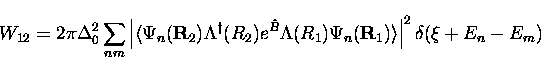(13)
where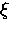is any small energy shift that may exist between initial and final sites, which must be taken up by differences in phonon energies, and the coupling to barrier fluctuations is characterized by the operator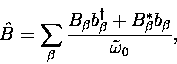(14)
where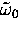is the frequency of the interstitial in its harmonic potential well, included as a normalization factor to make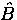dimensionless.

Expanding the square of the matrix element in Eq. (5.22) and writing the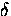-function in terms of its Fourier transform, we can write the hop rate as an integral over time

The rest of the problem is in the calculation of F1(t), which contains all the physics.

Expanding each part in terms of the creation and annihilation operators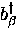,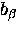,(for which we have the commutator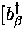,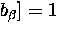since phonons are bosons)

where the factor eB0 is a scalar resulting from the commutators which arise in the product of these operators, in which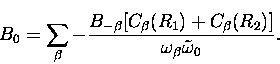(15)

Doing the same calculation with the second set of time-dependent operators, [with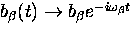]and then calculating the expectation value of the product of all six operators gives the result

which now becomes a function of temperature through the temperature dependence of the phonon population.

with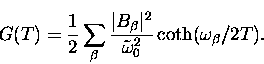(16)

Introducing two more sums over the phonon spectrum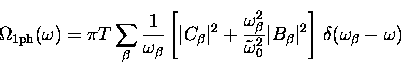(17)(18)

results, with more algebra, in the general expression

By shifting the contour on the complex plane, this is

Due to the presence of the oscillating terms, e-F1(t) is peaked about t=0, and so makes the largest contribution to the integral in Eq. (5.25) near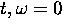. For small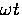and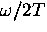several approximations can be made to further simplify these expressions, leading to an expression that yields its (approximate) temperature dependence in a more intuitive way.

and

Using these approximations and defining the following functions enables us to write an expression for the hop rate in which all the sums over the phonon population have been integrated out.

In terms of these functions we obtain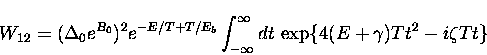(19)

This integral can be solved exactly, giving a simple expression for the hop rate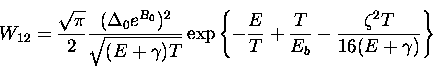(20)

If the coupling to barrier fluctuations turns out to be negligible (that is, letting all the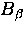's go to zero) then we recover the familiar expression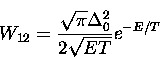(21)
giving the hop rate of the particle and associated polaron in the absence of any effect of barrier height fluctuations, which was originally obtained by Flynn and Stoneham. Here, E is just the barrier height which would exist between two adjacent polaron-deformed sites.

The motivation for making all of these approximations was to allow one to write a simple expression for the hop rate. It turns out that the existence of an upper cut-off frequency (essentially the Debye frequency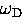) in the sums over the phonon modes makes it possible to calculate the integrals in the general form, Eq. (5.35), by numerical methods without making any further approximations. This approach is taken in the analysis of Mu diffusion in solid Xe.Next: 5.5 Quadratic Coupling: Two-Phonon Up: 5 Quantum Diffusion I: Previous: 5.3 Linear Coupling; One-Phonon